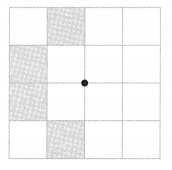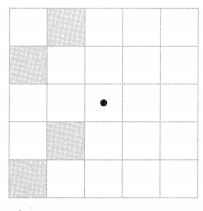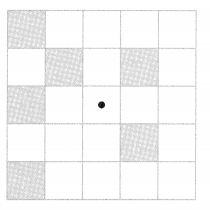# Math in Focus Grade 4 Chapter 13 Practice 3 Answer Key Making Symmetric Shapes and Patterns

This handy Math in Focus Grade 4 Workbook Answer Key Chapter 13 Practice 3 Making Symmetric Shapes and Patterns detailed solutions for the textbook questions.

## Math in Focus Grade 4 Chapter 13 Practice 3 Answer Key Making Symmetric Shapes and Patterns

Each figure below is half of a symmetric shape with the dotted line as a line of symmetry. Complete each symmetric shape.

ExampleQuestion 1.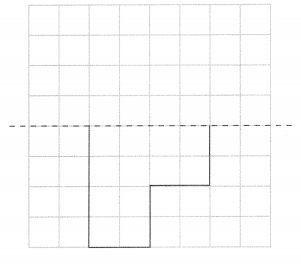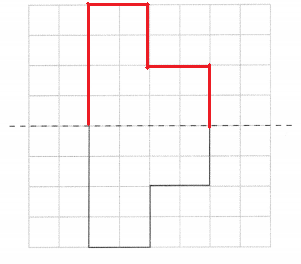Question 2.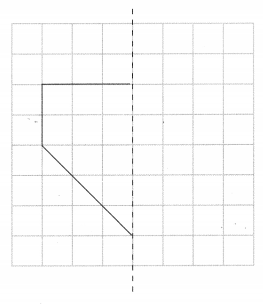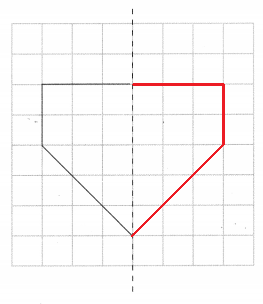Question 3.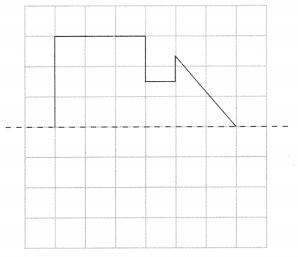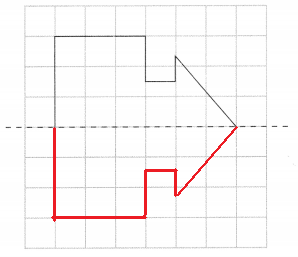Each figure below is half of a symmetric shape with the dotted line as a line of symmetry. Complete each symmetric shape.

Question 4.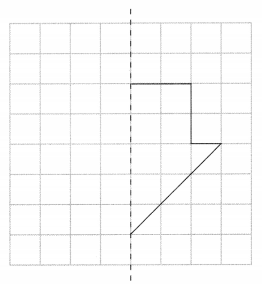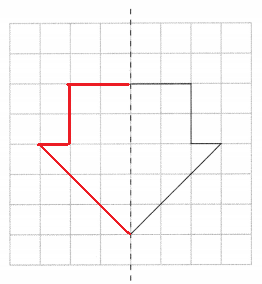Question 5.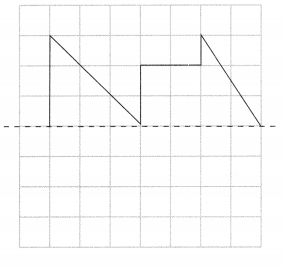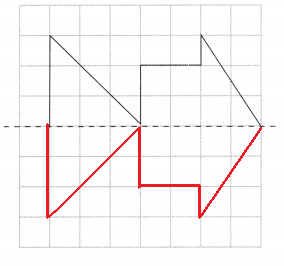Shade four more squares in each figure so that the pattern of shaded squares has rotational symmetry.

Example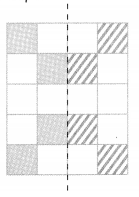Question 6.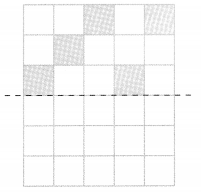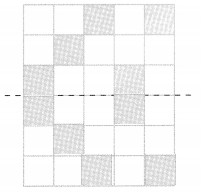Question 7.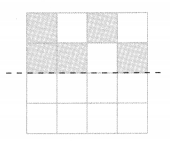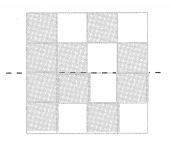Question 8.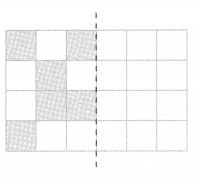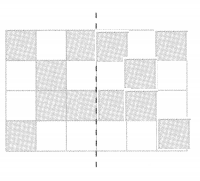Shade four more squares in each figure so that the pattern of shaded squares has rotational symmetry.

Example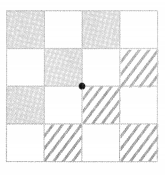Question 9.